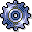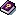Target / Actual / Production VariancesUse

Report with which you can analyze the results ofVariance Calculation in the following application components of Product Cost Controlling using product drilldown:

• Product Cost by Period
• Product Cost by Order

The reporting time frame can be cumulative or limited.

This report shows the results of variance calculation. The results are dependent on which target version was specified at report call. (see also:Types of Variance Calculation,Target Cost Versions in Variance Calculation)

You can call up a detailed report for the variance categories to investigate the production variances in more detail. There are two scenarios for the interpretation of the data:

• Scenario 1: Standard costs, alternative material cost estimate, preliminary order cost estimate versus (if necessary, net) actual costs

• Scenario 2: Standard cost estimate for the material versus planned costs for the order

Standard cost estimate for the material versus planned costs for the order corresponds to target cost version 2 (planning variance)

Structure

Data Displayed

Scenario 1: Standard costs, alternative material cost estimate, preliminary order cost estimate versus (net) actual costs

The data is based on key figures and/or cost elements:

• Control costs = Actual costs – WIP – Scrap variance

Scenario 2: Standard cost estimate versus preliminary order cost estimate (planning variance)

The data is based on key figures and/or cost elements:

• Target costs = values of the standard cost estimate adjusted to plan quantity of the order (planned lot size of the manufacturing order)
• Actual costs
• Variances = Calculated variances between preliminary order cost estimate and target costs calculated on basis of standard cost estimate
• Scrap variance (has no relevance for planning variances)
• Work in process (WIP) (has no relevance for planning variances)
• Control costs = planned costs for the order

• Object information
• Reporting time frame

Integration

Prerequisites

Make sure that the variances have been calculated for the specific target cost version in the relevant reporting time frame. Reports of summarized analysis require that a data collection run be executed beforehand.

Notes

When you interpret this report, note whether objects with a settlement rule with only one settlement type PER (periodic) or FUL (full settlement) are included, or whether you are accessing a report in summarized analysis in which objects have been summarized with both settlement types.

Settlement type "Periodic"

The following interpretations for each period under consideration apply only to a report for an object with the settlement type periodic:

Variances = Target costs – Control costs

Control costs = Actual costs - WIP - Scrap variances (only for SAP standard target cost versions 0,1, and 3)

In a report with or i ginal cost elements, work in process is displayed in its source cost elements. The cost estimate used to calculate the source cost element is the same as the one used to valuate work in process at target costs. You determine which cost estimate is used by determining the valuation variant for work in process and scrap (see also:Work in Process in Product Cost by Period).

Settlement type "Full Settlement"

Note the following points with objects that have the settlement type full settlement:

• Work in process is not displayed in its source cost elements, because no target costs are calculated on the basis of another cost estimate for valuation of work in process. Rather, work in process corresponds to the balance of the order for which final delivery has not yet taken place (see also:Work in Process in Product Cost by Order). Work in process is updated under results analysis cost elements. To analyze work in process, use the report Work in Process.
• Target costs and variances are only calculated and updated cumulatively in the period in which the status DLV (Delivered) or TECO (Technically completed) has been reached for the manufacturing order.

Notes on Summarized Analysis with Product Drilldown

Work in process is derived from the results analysis cost elements for both objects with settlement type "Periodic" and objects with settlement type "Full settlement". If the results analysis cost elements are not assigned in the cost component structure for product drilldown, the work in process appears in the cost component Other (if defined) or as not assigned.

Notes on Detailed Reports with Original Cost Elements

Scenario: variances have not yet been calculated

• Target cost calculation is initiated dynamically
• No variances are calculated
• No data is updated

This option enables an ad hoc target/actual comparison for a single object.

Scenario: variances have been calculated

• Target costs according to variance calculation
• Variances according to variance calculation